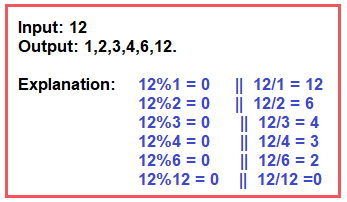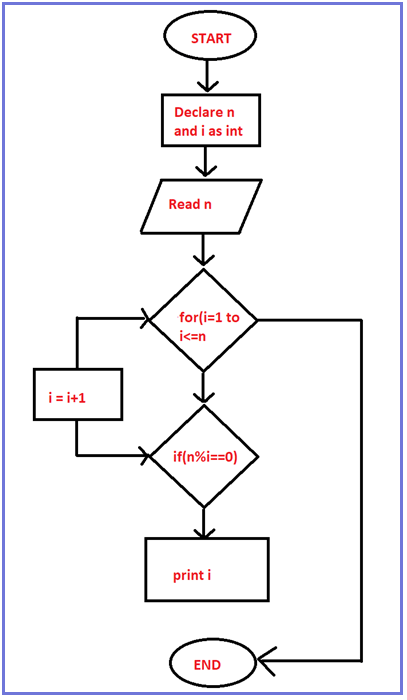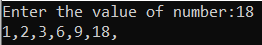# How to Find all Factors of a Given Number in C#

## How to Find all Factors of a Given Number in C# with Examples

In this article, I am going to discuss How to Find all the Factors of a Given Number in C# with Examples. Please read our previous article, where we discussed How to Convert Kilogram to Gram and Vice Versa in C#.

##### What is Factor?

In mathematics, a number or algebraic expression that divides another number or expression evenly i.e., with no remainder. For example, 3 and 6 are factors of 12 because 12 ÷ 3 = 4 exactly and 12 ÷ 6 = 2 exactly. The other factors of 12 are 1, 2, 4, and 12.

###### Example:##### Algorithm to Find Factors of a Given Number

Step1: Take the input value and store it into a variable called number.
Step2: Declare a variable named factor and initialize it with 1 because for all the numbers 1 will be one of the factors.
Step3: Then apply the Modulus operator number with the factor. If it equals 0. Print the value of factor value as output. And increment the value of the factor.
Step4: Repeat step 3 until the factor is less than or equal to the number.

###### Flow Chart##### Example: C# Program to Find all the factors of a number.

The following C# Program will print all the factors of a given number.

```using System;
namespace DotNetTutorials
{
public class PrintFactorials
{
static void Main(string[] args)
{
Console.Write("Enter the value of number:");

int factor;
for (factor = 1; factor <= number; factor++)
{
if (number % factor == 0)
{
Console.Write(factor + ",");
}
}